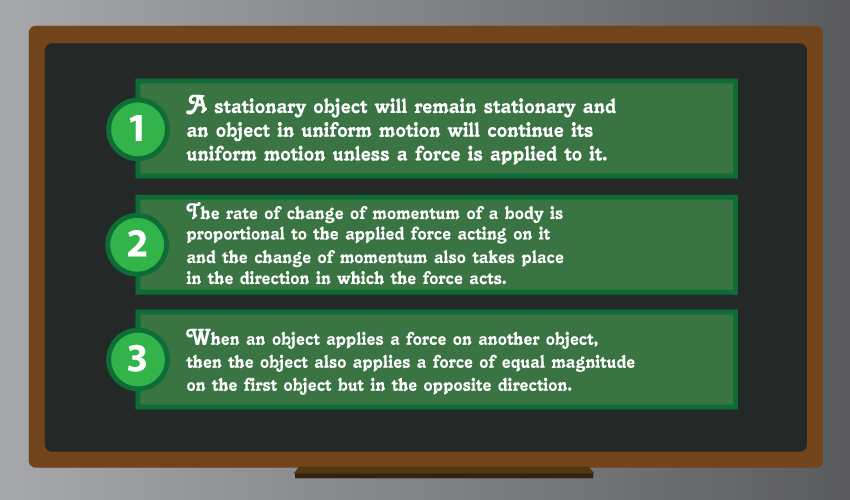# What are Newton’s Laws of Motion?Newton’s Laws of Motion basically explain the constant velocity of an object unless acted upon by a force, the mass of the object multiplied by the acceleration of that object, and the equal and opposite reaction of the force of that object.

We all are familiar with Newton’s laws of motions, at least with the phrase if not what they are. Sir Isaac Newton was a genius man who was the first person to figure out the existence of gravity. Remember the story about an apple falling on the ground rather than going up? His laws and theories are applied in many fields of life and they actually laid the very foundations of physics about 300 years ago. When he was only 23, he promulgated his theory of universal gravity which was a big discovery in the world of science.

Newton’s laws of motion were published in one of his most renowned works "Principia Mathematica Philosophiae Naturalis", popularly known as "Principia". These laws are incorporated in the very initial stages of the formal education in schools all over the world.

There are three laws of motion which are very basic to understand as they can be found in real life in everything that happens around us and all the things that we do.

## 1st Law

The first law of motion is also called the law of inertia. As per the first law, the position of an object changes only when a force is applied to it. This force must be unbalanced, as in it shouldn’t cancel out the force exerted by the object itself otherwise it wouldn’t change its position. So for an object to move there has to be an unbalanced force to make it displace, otherwise, it will remain at rest. And, if it’s already at rest then an unbalanced force must be exerted on it to make it move. For example, if we leave an object in space it won’t stop moving until it is hit by an object say meteorite. Or let’s take a closer example; when we drive, the car will keep going unless we intend to stop it by pressing the brake or if it, unfortunately, hits a tree or a wall. Simply put, there needs to be an external force to change the object’s position.

## 2nd Law

The second law is relatively more interesting because it introduces another factor to the equation i.e. mass. Mass is the overall quantity that a body contains. In order for an object to move, we need to apply an unbalanced force on it which must be according to the mass of the object. It’s very simple. It takes more force to push a gallon of water than pushing a 500 ml bottle. Another example can be that an airplane requires more force to move than a car or a truck because it’s greater in mass.

## 3rd Law

The third law is really fun to rote learn to be very honest. It says: “for every action, there’s an equal and opposite reaction”. Let’s say a person throws a ball towards a wall, it bounces back. We see it bouncing back right where it’s thrown. This is what the third law states. Whenever a force is exerted on something, the thing exerts back another force with the same momentum. But is the velocity same? It can be but its direction will be opposite. When we blow a balloon and release it, the air escaping is one force and the balloon flying up is its opposite reaction. When we jump on a trampoline, we exert a force on it, which in turn pushes us upwards. All these examples show what Newton said in his last third of motion.

## Conclusion:

Newton’s Laws of Motions are still regarded as the three fundamental laws of physics. The foundation of classical mechanics was built on these three laws. In a nutshell, the Law of Inertia, the Law of Mass and Velocity, and the Law of Equal and Opposite Reaction are the three laws of motion.